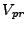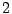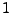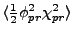Next: dvdf(int fld, INDEXLIST) Up: Writing a Model File Previous: modelinitialize()

### potential_energy(int term, float *field_values)

This function returns a term from, averaged over the lattice. You can choose to break the potential into separate terms in any way you wish. You can, for example, simply call the whole thing one term. The only difference this will make is that if you output the components of energy (see section 5.5) each term will be output separately. The number of terms must be given as the value of the constant num_potential_terms. For example, in the TWOFLDLAMBDA model this constant is set to. If the argument term isthe function returnsand if term isit returnswhere angle brackets denote lattice averages.

The second argument to the potential energy function is a pointer to a list of average field values. Normally during the run this pointer will be passed in as NULL, in which case the function does the grid averages described above. When setting the initial conditions, however, the program will call this routine before the field values on the lattice have been set. In this case it will pass in an array of values representing the initial homogeneous values of the fields. If the pointer that is passed in is not NULL then the function will calculate the potential terms using these values rather than by averaging over lattice values. The implementation of these options should be clear in the TWOFLDLAMBDA model file.Next: dvdf(int fld, INDEXLIST) Up: Writing a Model File Previous: modelinitialize()